Courses

# Test: Lines And Lines

## 10 Questions MCQ Test Mathematics (Maths) for Class 2 | Test: Lines And Lines

Description
This mock test of Test: Lines And Lines for Class 2 helps you for every Class 2 entrance exam. This contains 10 Multiple Choice Questions for Class 2 Test: Lines And Lines (mcq) to study with solutions a complete question bank. The solved questions answers in this Test: Lines And Lines quiz give you a good mix of easy questions and tough questions. Class 2 students definitely take this Test: Lines And Lines exercise for a better result in the exam. You can find other Test: Lines And Lines extra questions, long questions & short questions for Class 2 on EduRev as well by searching above.
QUESTION: 1

### What is wrong with this number line?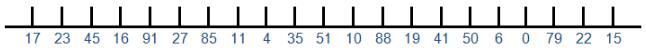Solution:

Saying the numbers aloud will sound all wrong!

QUESTION: 2

### What would be the last number of this part of the number line?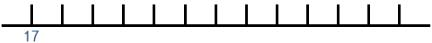Solution:

Pointing to each mark and saying the number is helpful

QUESTION: 3

### Which numbers are missing from this number line?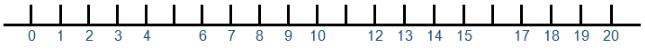Solution:

Say the numbers as you count up

QUESTION: 4

Which numbers are missing from this number line?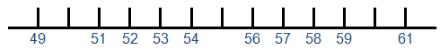Solution:

Look at the numbers which come before and after the blank spaces

QUESTION: 5

Which numbers are missing from this number line?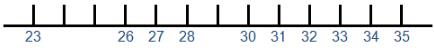Solution:

Look at the numbers which come before and after the blank spaces

QUESTION: 6

Which other numbers might you find on this part of the number line?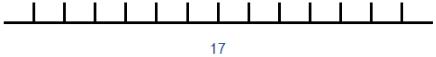Solution:

Count forwards and backwards from the center number

QUESTION: 7

Which numbers are missing from this number line?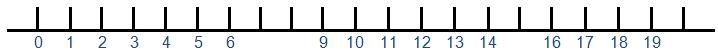Solution:

QUESTION: 8

Which number would be found at the beginning of this number line?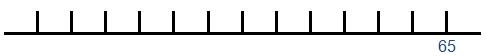Solution:

Pointing and counting down aloud may help

QUESTION: 9

What is special about all the numbers missing from this number line?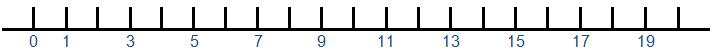Solution:

These numbers are in the two times table

QUESTION: 10

What other numbers might you find on this part of the number line?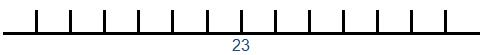Solution:

Count forwards and backwards from the center number